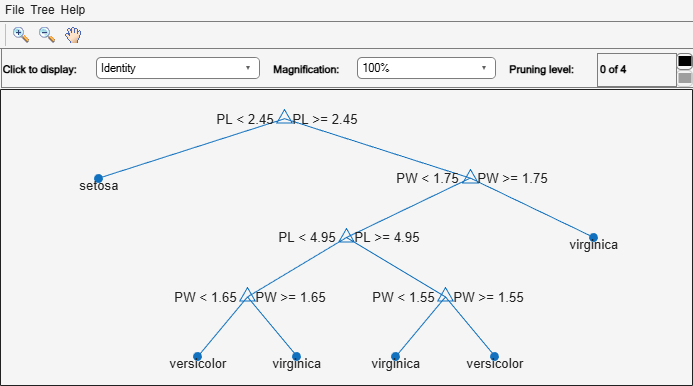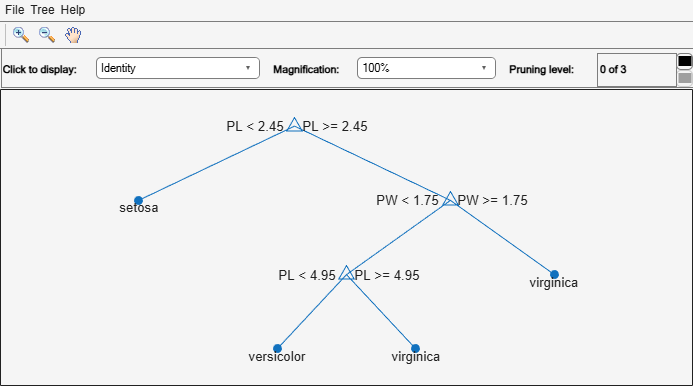# prune

Produce sequence of classification subtrees by pruning

## Syntax

``tree1 = prune(tree)``
``tree1 = prune(tree,Name,Value)``

## Description

````tree1 = prune(tree)` creates a copy of the classification tree `tree` with its optimal pruning sequence filled in.```

example

````tree1 = prune(tree,Name,Value)` creates a pruned tree with additional options specified by a `Name,Value` pair argument. You can only use one option at a time.```

## Examples

collapse all

Construct and display a full classification tree for Fisher's iris data.

```load fisheriris; varnames = {'SL','SW','PL','PW'}; t1 = fitctree(meas,species,'MinParentSize',5,'PredictorNames',varnames); view(t1,'Mode','graph');```Construct and display the next largest tree from the optimal pruning sequence.

```t2 = prune(t1,'Level',1); view(t2,'Mode','graph');```## Input Arguments

collapse all

Classification tree, specified as a `ClassificationTree` model object produced by the `fitctree` function.

### Name-Value Arguments

Specify optional pairs of arguments as `Name1=Value1,...,NameN=ValueN`, where `Name` is the argument name and `Value` is the corresponding value. Name-value arguments must appear after other arguments, but the order of the pairs does not matter.

Before R2021a, use commas to separate each name and value, and enclose `Name` in quotes.

Example: `tree1 = prune(tree,'Level',1)`

Pruning cost, specified as a numeric scalar. When this option is specified, `prune` prunes `tree` to the specified value of the pruning cost.

Pruning level, specified as a numeric scalar from `0` (no pruning) to the largest pruning level of this tree `max(tree.PruneList)`.

Branch nodes to turn into leaf nodes, specified as a numeric vector with elements from `1` to `tree.NumNodes`. Any `tree` branch nodes listed in `Nodes` become leaf nodes in `tree1`, unless their parent nodes are also pruned.

## Tips

`tree1 = prune(tree)` returns the decision tree `tree1` that is the full, unpruned `tree`, but with optimal pruning information added. This is useful only if you created `tree` by pruning another tree, or by using the `fitctree` function with pruning set `'off'`. If you plan to prune a tree multiple times along the optimal pruning sequence, it is more efficient to create the optimal pruning sequence first.

## Version History

Introduced in R2011a